# What Are Circles Used For? (12 Real Life Uses Of Circles)

Circles are used often in mathematics for word problems in geometry or trig functions (unit circle).  However, circles are also vital for many purposes in everyday life.

So, what are circles used for?  Circles are used for various applications in architecture, gardening, graphics, infrastructure, and transportation. For example, an arch in a building is part of a circle. We use circles to figure out how far apart to plant trees. Circles also help us to find the distance a car has driven.

Of course, circles have many more applications in real life, from the design of everyday objects (like cups, clocks and hoses) to circular tracks for walking and running.

In this article, we’ll talk about 10 important real life uses of circles.  We’ll also give some examples so you can see the numbers at work.

## What Are Circles Used For? (10 Real Life Uses Of Circles)

Circles have many applications in everyday life, from architecture to track & field.  Here are 12 ways that circles are used in real life:

• Architecture
• Calculating Volume (Cones & Cylinders)
• Clocks
• Cups
• Gardening (Plants & Trees)
• Gears
• Graphics (Rotations)
• Hoses & Pipes
• Manhole Covers
• Odometer (Calculating Distance)
• Painting With A Roller
• Tracks (For Walking & Running)

Let’s take a look at each of these in turn, starting with architecture.

### Architecture

Circles are used in architecture for many purposes.

For one thing, circular columns (pillars) are often used to support roofs.  A round column can carry more load than a square column, since the weight is distributed evenly at all points along the circle’s circumference.

Some arches are also constructed using semicircles, such as the one pictured below.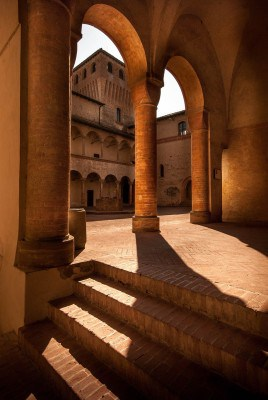Rounded arches use part of a circle in their design. Arches were used in ancient times to support aqueducts and other structures.

### Calculating Volume (Cones & Cylinders)

Since circles are used in the design of so many objects, they are also helpful in finding the volume of those objects, including cylinders and cones.

#### Example 1: Volume Of A Cylinder

Let’s say that we have a cylinder with a height of 10 inches and a radius of 6 inches (so the circular base of the cylinder is 12 inches or 1 foot across at its widest point).

The volume of a cylinder is given by the formula

• V = πR2H

where V is the volume, R is the radius of the circular base, and h is the height.  Using H = 10 and R = 6, we get:

• V = π(6)210
• V = 360π
• V = 1131 cubic inches

So the volume of this cylinder is 1131 cubic inches.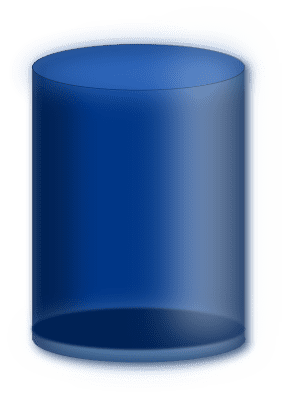To find the volume of a cylinder, we need its height and the area of its circular base, which requires a radius.

#### Example 2: Volume Of A Cone

Let’s say that we have a cone with a height of 15 inches and a radius of 9 inches (so the base of the cone is 18 inches or 1 foot across at its widest point).

The volume of a cylinder is given by the formula

• V = πR2H / 3  [one third the volume of the cone with the same circular base and height]

where V is the volume, R is the radius of the circular base, and h is the height.  Using H = 15 and R = 9, we get:

• V = π(9)215 / 3
• V = 1215π / 3
• V = 1272 cubic inches

So the volume of this cone is 1272 cubic inches.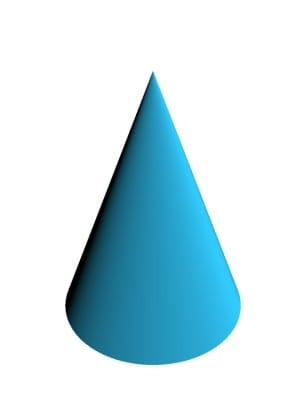To find the volume of a cone, we need its height and the area of its circular base, which requires a radius.

#### Example 3: Finding Radius Of A Cylinder for A Given Volume & Height

Given a cylinder with a volume of 3142 cubic inches and a height of 10 inches, we can solve for the radius of the circular base using the equation for the volume of a cylinder:

• V = πR2H
• 3142 = πR2(10)
• 314.2 = πR2
• 100 = R2
• 10 = R

So, the radius of the cylinder would need to be 10 inches to give the desired volume of 3142.

### Clocks

The shape of a clock is often a circle because of the hands.  The hour, minute, and second hands are all attached to the center of the clock.

The end of each hand moves around in a circle whose radius is the length of the hand.  So, it makes the most sense for a clock to be round.

Even for a clock that is square (or some other shape), the hands will still trace out circles.

### Cups

Imagine trying to drink or pour from a cup shaped like a triangle, square, or other shape.  The corners would make it difficult to do, and you would probably spill a lot more often.

In addition, cups and bowls with corners are more difficult to clean, since food or drink could dry up and get stuck in the corners.

Circular cups are also easier to hold, since you do not have a corner digging into your hand as you would with a square cup.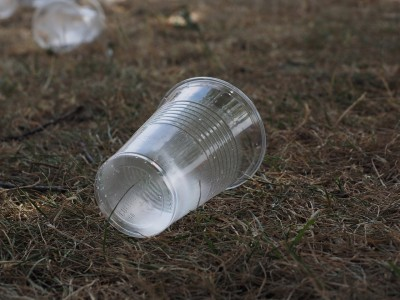A circular cup is easier to hold, and it won’t spill as much when drinking from it (compared to a square cup). It will also be easier to clean.

### Gardening (Plants & Trees)

Circles are also useful in gardening when you are trying to figure out where to put your plants.  If a plant needs 1 foot of space around it in every direction, then each plant needs a circle with a radius of 1 foot.

Circles also help to describe how trees grow.  The roots will spread out from the base of the tree in all directions in a circle.

Usually, the branches above ground grow about as far out as the roots.  So, you can use the branches as an indication of how far from the tree to water.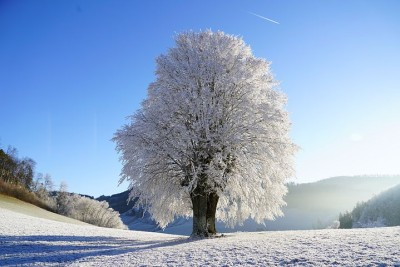We can use circles to tell us how far apart to plant trees to give each one enough space to grow.

### Gears

A gear is not exactly the shape of a circle.  Instead, it is a modified circle with “teeth” that catch the teeth on another gear so that one causes another to turn.

We can use the circumference and rotation speed of a gear to figure out how long it takes to complete one full revolution.  We can also use the number of teeth to figure out how much the gear rotates each time a gear moves.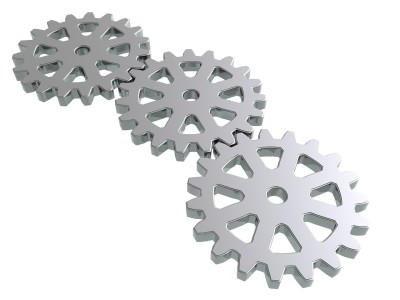A gear is a modified circle with teeth that is used in cars and other machinery. When one gear turns, its teeth cause other adjacent gears to turn as well.

### Graphics (Rotations)

Anytime you see a rotating object in a movie or video game, you are seeing circles at work.  There is a fixed center point for the rotation, and the distance from the center to the object is the radius of the circle.

### Hoses & Pipes

Hoses are round because they are easier to hold.  Pipes are round because square pipes could get debris caught in the corners.

A circular shape for hoses and pipes also means a stronger shape, which allows for more pressure inside without causing much distortion.

### Manhole Covers

Manhole covers are round because this ensures that the cover can never fall into the hole.  No matter which way you turn a circular manhole cover, its widest point (the diameter) cannot pass through the hole, since its width is slightly less than the diameter.

On the other hand, a square manhole cover could fall into the hole.  If each side is 24 inches, then the diagonal is 24√2 or about 34 inches.

If you rotate the square manhole cover by 45 degrees, it will be able to clear the diagonal of the hole (24 inches versus 34 inches, or 10 inches of clearance).

### Odometer (Calculating Distance)

You can also use circles for an odometer to calculate the distance that a car travels.  This is based on the circumference of the wheel and the number of revolutions.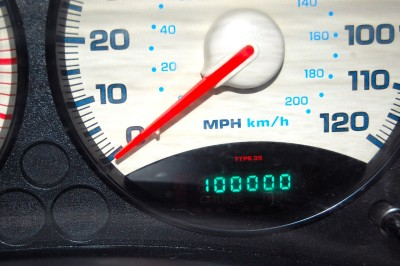An odometer measures the distance that a car travels, based on the size of the wheels and the revolutions completed.

#### Example: Using Circles To Calculate Distance Traveled By A Car

Let’s say that your car has wheels with a diameter of 18 inches (so the radius is 9 inches).  If you travel at 1,000 RPM (revolutions per minute) for one hour, how far have you gone?

First, we find the circumference of the circular wheel.  The formula for circumference is

• C = 2πR

where C is the circumference and R is the radius.  Since the radius of the wheel is R = 9, we get a circumference of:

• C = 2π(9)
• C = 56.6 inches

This means that the car will travel 56.6 inches every time the wheel completes one revolution.

Since the car is running at 1,000 RPM, then it is completing 1,000 revolutions in one minute.  There are 60 minutes in an hour, so the car is completing 1,000*60 = 60,000 revolutions per hour.

So, the car has traveled a distance of (60,000 revolutions)*(56.6 inches per revolution) = 3,392,920 inches.  Dividing by 12 gives us 282,743 feet.

Dividing by 5,280 gives us 54 miles.  So, the car was traveling at an average speed of 54 miles per hour during the trip.

### Painting With A Roller

Painting a wall with a roller makes the work go much faster.  The shape needs to be round for the roller to work and for the paint to be applied smoothly and uniformly.

A larger roller can hold more paint before you need a refill, but too much would make the roller heavy and cause more spills.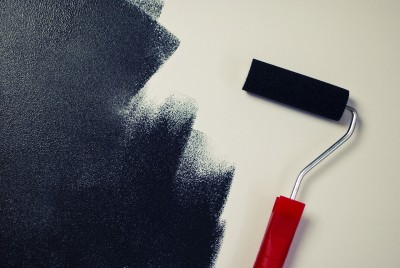A paint roller has a circular shape so that it can roll smoothly and apply paint evenly.

### Tracks (For Walking & Running)

When running around a circular track, the circumference (perimeter) tells you how much distance you cover every time you go around.

#### Example: Distance Around A Circular Track

You want to know the distance around a circular track at a college.  The track is 400 feet from the center to an edge (radius = 400 feet).

Then the perimeter (circumference) of the circular track is:

• Circumference = 2*π*R [R = radius of circle]
• Circumference = 2*π*400
• Circumference = 800*π
• Circumference = 2513 feet

So, one time around the track is 2513 feet – just under a half of a mile (which is 2640 feet).

## Conclusion

Now you know some of the uses of circles in real life, along with how to do some of the calculations for those applications.

You can learn about uses of hexagons (and appearances in nature) here.

You can learn about arc length of a circle here.

You can learn about area of a circular sector here.

You can find out more about squares and circles here.

You can learn about various uses of algebra in real life here.

You can learn about the history of pi and how it is connected to circles here.